# Introduction

## Integrals class 12 of Class 12

### Definition

If f and F are functions of x such that F′(x) = f(x) then the function F is called a primitive or an indefinite integral of f with respect to x and is expressed as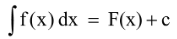where c is a constant of integration.

f(x) then possesses infinitely many antiderivatives, all of them being contained in the expression F(x) + c where c is a constant.

From the geometric point of view, an indefinite integral is a collection (family) of curves, each of which is obtained by translating one of the curves parallel to itself upwards or downwards.

### Expression Substitution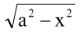x = a sin θ  or a cos θx = a tan θ  or a cot θ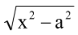x = a sec θ or a cosec θ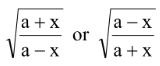x = a cos θ  or a cos 2 θ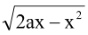x = a (1 - cos θ)

## Critical Points

The points on the curve y = f(x) at which dy/dx = 0 or dy/dx does not exist are called critical points.